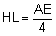< PREVIOUS PROBLEM  |  NEXT PROBLEM > Problem 50. Triangle with Equilateral triangles. Level: High School, SAT Prep, College In the figure below, equilateral triangles ABD and BCE are drawn on the sides of a triangle ABC. F, G, and H are the midpoints of AD, CE, and AC respectively. HL and FG are perpendicular. Lines FH, CD, HL,  and FG are cut by line AE at J, O, P, and N respectively. Lines GH, HL, and FG are cut by line CD at K, Q, and M respectively. Prove the following: AE = CD The measure of angle DOE is 120º FH = GH The measure of angle FHG is 120º mÐHFG = mÐHGF = 30º OB is the bisector of ÐDOE mÐOBC = mÐOEC = mÐHGC mÐADO = mÐABO = mÐAFH OD is the bisector of ÐAOB OE is the bisector of ÐBOC mÐAOB = mÐBOC = 120º mÐCMG = mÐANF = 30º OB and FG are perpendicular Triangle OPQ is equilateral Triangle JPH is equilateral Triangle HKQ is equilateral HJ = HK + OPHints: See Geometry Help
 Home | Geometry | Problems | Open Problems | 10 Problems | 41-50 | Email | By Antonio Gutierrez Post or view a solution to the problem 50